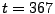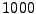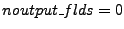Next: Run Parameters Up: LATTICEEASY http://www.science.smith.edu/departments/Physics/fstaff/gfelder/latticeeasy Previous: Macros for Referencing Field

# Output

This section explains the output of LATTICEEASY. Having calculated the evolution of a set of fields the program has many functions for calculating and saving derived quantities about those fields. These quantities include spatial means and variances, spectra, histograms, and more. The way this works in the program is the following. Every set number of time steps (described below) the program calls the function save(), which in turn calls a series of functions designed to calculate and save certain quantities. Which of these output functions gets called is determined by a set of parameters set in the file parameters.h. For example the parameter smeansvars determines whether means and variances should be calculated for a particular run.

The functions for means and variances and for recording values of the scale factor and its derivatives are called each time save() is called. The other functions, for saving spectra, energy density, histograms, slices through the grid, and images of the grid, are calculated less often. The parameter checkpoint_interval sets how often (in program time units) to call these functions. This same parameter also controls how often the output file buffers are flushed. So for example ifandand the program is interrupted atthen the program should have saved a complete grid image atand all output files should have been written at that time, so the program may easily be resumed from that point without any loss of data. The function model_output() is called every time save() is called, but it is passed a parameter indicating whether infrequent calculations are being performed. This way if you design model specific output functions you can decide whether to calculate them frequently or infrequently.

There is another parameter called noutput_flds that controls how many fields should be included in the output. Aside from the energy density (which must include all fields in order to check energy conservation) and two dimensional histograms (which use an explicit list of fields given in parameters.h) the output functions only perform calculations for the first noutput_flds fields. This is useful if, for example, you are doing a run with several fields that you expect to act nearly identically. If noutput_flds is set to zero then all fields will be output.

How often save() is called is set by the parameter noutput_times, which specifies how many times during the run the save() function should be called. We have foundto be a convenient number that generally gives reasonably high resolution output but doesn't create awkwardly large output files. For runs for which high resolution is requiredoutputs (or more) might be appropriate.

There is another output function that is not called by save(), but directly by the main program. This function, output_parameters(), is called only at the beginning and end of a run. It outputs a description of the model being used, the values of the run parameters, the date and time at the beginning and end of the run, and the total elapsed clock time during the run.

The names of the output files are all given extensions that depend on a couple parameters. The default extension is ``_0.dat". For example the file that stores the field means will be called means_0.dat. If, however, you are continuing a previous run (see section 4.1.2) and you elect to create new output files rather than appending data to the old ones then the new files will be given the extension ``_1.dat". If you continue that run the new extension will be ``_2.dat" and so on. Note, however, that if you set the string alt_extension in parameters.h to be anything other than empty then it will be used as the extension for all output files no matter what.

The function output_initialize() is called at the beginning of the run to set the default extension and the number of output fields. (The number of output fields must be reset to the total number of fields if.)

The field means, variances, histograms, and slices are all set to multiply the field values being output by a rescaling value. By default this value is simply one, meaning the values being output are the program field values. This option is included, however, so that you can calculate a rescaling that you wish to use in your model. This calculations could be done once in the run or separately at each output time. For example, you could use this to reverse the program rescalings and instead output field values in physical units. If you do set the rescaling once you should do so in model_initialize(), whereas if you want to reset it repeatedly as the run progresses you should do it in the model_output() function described below.

Each of the functions that generates output is described in more detail below. Each section below gives the name of an output function, the name(s) of the files it creates, the values of its outputs, and any other necessary information. With one exception, all of the functions described here are contained in the file output.cpp. That one exception is the function model_output(), which is a function in the model file that allows you to implement outputs specific to a particular model. In these sections we will refer to the output files with the extension ``_ext," which will typically be ``_0.dat" as described above.

SubsectionsNext: Run Parameters Up: LATTICEEASY http://www.science.smith.edu/departments/Physics/fstaff/gfelder/latticeeasy Previous: Macros for Referencing Field